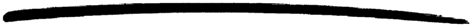# erl_internal

erl_internal

### MODULE SUMMARY

Internal Erlang Definitions

### DESCRIPTION

This module defines Erlang BIFs, guard tests and operators. This module is only of interest to programmers who manipulate Erlang code.

### EXPORTS

Types:

Name = atom()
Arity = integer()

Returns true if Name/Arity is an Erlang BIF which is automatically recognized by the compiler, otherwise false.

Types:

Name = atom()
Arity = integer()

Returns true if Name/Arity is an Erlang BIF which is allowed in guards, otherwise false.

Types:

Name = atom()
Arity = integer()

Returns true if Name/Arity is a valid Erlang type test, otherwise false.

Types:

OpName = atom()
Arity = integer()

Returns true if OpName/Arity is an arithmetic operator, otherwise false.

Types:

OpName = atom()
Arity = integer()

Returns true if OpName/Arity is a Boolean operator, otherwise false.

Types:

OpName = atom()
Arity = integer()

Returns true if OpName/Arity is a comparison operator, otherwise false.

Types:

OpName = atom()
Arity = integer()

Returns true if OpName/Arity is a list operator, otherwise false.

Types:

OpName = atom()
Arity = integer()

Returns true if OpName/Arity is a send operator, otherwise false.

Types:

OpName = atom()
Arity = integer()
Type = arith | bool | comp | list | send

Returns the Type of operator that OpName/Arity belongs to, or generates a function_clause error if it is not an operator at all.

stdlib 1.16.3